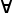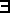# Maths - Predicate Logic

Predicate Logic (or also called Predicate Calculus) is the term for a formal and symbolic system of logic like first-order logic, second-order logic and so on.

It is defined over some (possibly infinite) set. It requires some form of assignment or relationship of the elements of the set to give true or false values.

### Predicate Logic

From site here :

A predicate is an expression of one or more variables defined on some specific domain. A predicate with variables can be made a proposition by either assigning a value to the variable or by quantifying the variable.

The following are examples of predicates :

• Let E(x, y) denote "x = y"
• Let X(a, b, c) denote "a + b + c = 0"
• Let M(x, y) denote "x is married to y"

## Terms

### Constants

Values have two values ether true of false, we may use alternative names as follows:

 true 1 T false 0 _|_

### Variables

A variable is a letter or symbol used to represent a value (an object, not a proposition). A predicate containing variables can be made into a valid proposition if the variables are either:

• assigned a value.
• or quanitified using a quantifier.

### Functions

Composite terms are formed by applying function symbols to other terms. Each function symbol has an 'arity' that is it takes in 'n' parameters and returns 'm' parameters.

## Quantifiers

 universal quantifier:for all existential quantifier:there exists (for some)

For universal quantifier we will use the notationx:σ.A(x) which is true if A(x) is true for every 'x' in type 'σ', where:

•is start of this universal quantifier syntax.
• x is a variable which ranges over given type.
• : means 'has type'.
• σ indicates the type.
• . seperator.
• A(x) is a predicate that is a function of x.

So,x:σ.A(x) = A(a) /\ A(b) /\ ...

where a:σ, b:σ ... are all objects in discourse.

Simarly for existential quantifier we will use the notationx:σ.A(x) which is true if A(x) is true for at least one 'x' in type 'σ'x:σ.A(x) = A(a) \/ A(b) \/ ...

where a:σ, b:σ ... are all objects in discourse.

#### Introduction RulesAx.A
(I) x not free in A
 A[t/x]x.A
(I)

#### Elimination Rulesx.A(x) A[x/t]
(E)
 [A] ...x.A B
B
(E) x not free in B

### Formulas

If x1, … ,xn are elements of the set and P is an n-place predicate symbol, then

P(x1, … ,xn) is an elementry formula

If A and B are formulas, then

• A /\ B
• A \/ B
• A ¬ B
• A ==> B
• A <=> B
•x A
•x A

are also formulas.

### Connectives

Commonly used logical connectives include:

• Negation (not) ( or ~)
• Conjunction (and) (/\,wedge, &, or )
• Disjunction (or) (/\ or vee )
• Material implication (if...then) (-> or supset)
• Biconditional (if and only if) (iff) (xnor) (<-> , equiv, or = )

## Programming

#### Formation Rules[x:A] ... A is a type P is a type
(x:A).P
(F) P is a type on the assumption that x is a variable of type A
 [x:A] ... A is a type P is a type
(x:A).P
(F) P is a type on the assumption that x is a variable of type A

#### Introduction Rules[x:A] ... .p:P
(λ x:A).p : (x:A).P
(I) x not free in A
 a:A p:P[a/x] (a,p):(x:A).P
(I)

#### Elimination Rulesa:A f: (x:A).P f a:P[a/x]
(E)
 p:(x:A).P fst p:A
 p:(x:A).P snd p: P[fst p/x]
(E) projections

#### Computation Rules((λ x:A).p a -> p[a/x] fst (p,q) -> p
snd (p,q) -> q